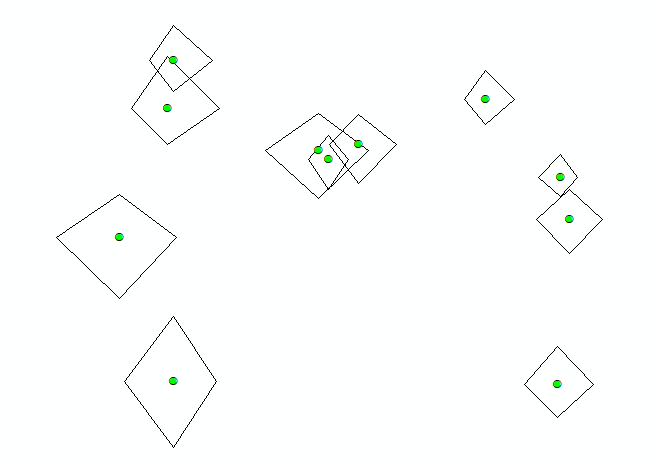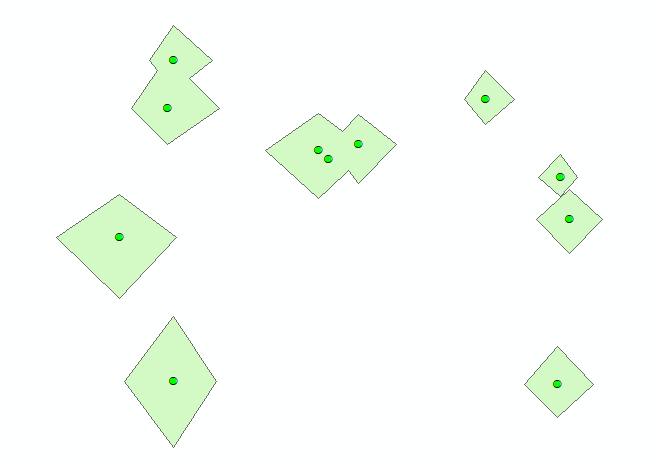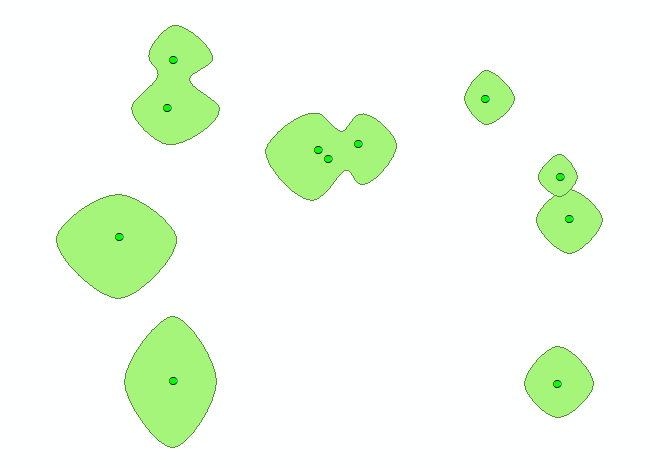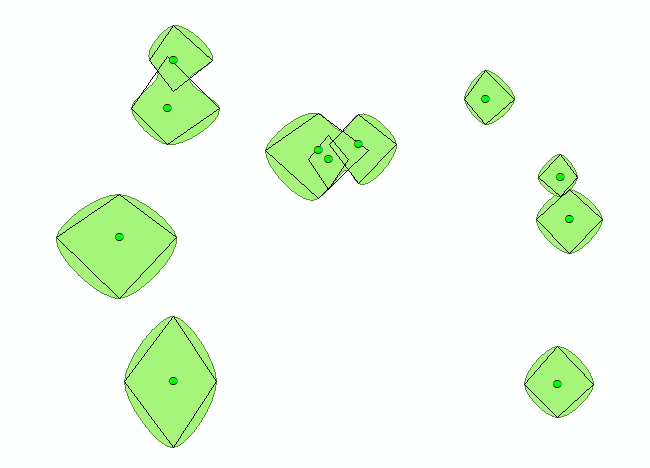Is it possible to create canopy spreads using precise distances and directions from the stem?

881
14
01-26-2018 03:53 AMby Anonymous User
Not applicable

Hi

Im currently creating Tree Constraints Plans in Arc but having trouble creating "representative canopy spreads". Our surveyors collect a North/South/East/West distance from a central point  and these distances are then used to depict the canopy spread.

We've been creating the canopies by calculating the average size using the measurements above then using this calculation to create a buffer - a nice circle and works very well. However we'd like to get a more representative shape as i'm sure you're aware tree canopies are rarely circular.

My initial thoughts were to place 4 points around the a center point at the distances and directions provided, then create a polygon using these points and finally smooth  the polygon to get an elliptical shape. However this is a long process, especially if we have over 200 trees to work with. That would mean plotting an additional 800 points at specific distances, then digitizing each individual canopy.....there must be a tool or a way of automating the process.

I've attached a couple of examples of the canopies. Example 1 is what we are aiming for example 2 is our current output.

Any ideas or suggestions would be hugely appreciated!

14 RepliesbyEsri Esteemed Contributor

Can you provide a sample of your data (point featureclass with the 4 measurements). I think this could be scripted, but I would have to take a look at the data to be sure.byEsri Esteemed Contributor

To show what is possible with some scripting I created a random dataset (would have been easier to do this on the actual data...) and this is what I ended up with:

def main():
import arcpy
import random

# output and intermediate fcs

# some settings for extent and number of trees (generated randomly)
xmin = -8397900
ymin = 693400
xmax = -8397300
ymax = 693800
trees = 75
min_dist = 5.0
max_dist = 15.0
directions = ['N', 'E', 'S', 'W']
sr = arcpy.SpatialReference(3857)

# generate the points and the NESW values into a list
feats = []
points = []
for i in range(trees):
x = random.randrange(xmin, xmax)
y = random.randrange(ymin, ymax)
pnt = arcpy.Point(x, y)
pntg = arcpy.PointGeometry(pnt, sr)
points.append(pntg)
feat = [pnt]
dist_range = random.randrange(min_dist, max_dist)
variation = dist_range / 2.0 + 1.0
for direction in directions:
feat.append(random.random()*variation+dist_range)
feats.append(feat)

# store the tree points as featureclass
arcpy.CopyFeatures_management(points, fc_pnt)

# step 1: create polygons using straight lines
polygons = []
for feat in feats:
pnts = []
lst_apply = [(0, 1), (1, 0), (0, -1), (-1, 0)]
for i in range(1, len(feat)):
tpl = lst_apply[i-1]
pnt = arcpy.Point(feat.X+tpl*feat[i], feat.Y+tpl*feat[i])
pnts.append(pnt)
pnts.append(pnts)
polygon = arcpy.Polygon(arcpy.Array(pnts), sr)
polygons.append(polygon)

# store the polygons from step 1
arcpy.CopyFeatures_management(polygons, fc_pol1)

# step 2: Use dissolve to union polygons with overlap
arcpy.Dissolve_management(in_features=fc_pol1, out_feature_class=fc_pol2,
dissolve_field="", statistics_fields="",
multi_part="SINGLE_PART", unsplit_lines="DISSOLVE_LINES")

# step3: use the smooth tool (Advanced license required) to smooth the polygons
# use BEZIER_INTERPOLATION to force the output line to go through the input vertices
arcpy.SmoothPolygon_cartography(in_features=fc_pol2, out_feature_class=fc_pol3,
algorithm="BEZIER_INTERPOLATION", tolerance="0 Meters",
endpoint_option="FIXED_ENDPOINT", error_option="NO_CHECK")

if __name__ == '__main__':
main()

So the first part is just to create the random data and will not be relevant for you. This should be replaced with some code to read out the points and obtain the N, E, S, W values from the attributes (that is the part up to line 39). On line 41 the actual code starts to read out the data and create polygons, connecting the measurements N, E, S, W relatively to the tree point:The second step (starting at line 57) will dissolve the overlapping tree polygons:The third step (see line 64) requires an advanced license smooths the polygon.It uses the BEZIER_INTERPOLATION setting which will force the polygons to go through the original vertices as you can see here:New Contributor II

Xander Bakker‌ I have exact same issue with some of our data. I have tried to use your code to create and smooth the polygons to visualise tree canopies, but it doesn't seem to be working. I have attached a sample of our data. Any help would be much appreciated.byEsri Esteemed Contributor

It probably doesn't work since you the code creates random points on the fly, since I didn't have any data to test it on. Now that you shared your data, here is a small part of the result:The code that I used:

def main():
import arcpy
import random

# output and intermediate fcs
fc_pnt  = r'C:\GeoNet\TreeElipse\tree_data_sample.gdb\tree_data'
fc_pol1 = r'C:\GeoNet\TreeElipse\tree_data_sample.gdb\canopy_squares_v01'
fc_pol2 = r'C:\GeoNet\TreeElipse\tree_data_sample.gdb\canopy_squares_diss_v02'
fc_pol3 = r'C:\GeoNet\TreeElipse\tree_data_sample.gdb\canopy_squares_diss_smooth_v02'

# flds

# some settings
sr = arcpy.Describe(fc_pnt).spatialReference

# generate the points and the NESW values into a list
flds = ('SHAPE@', fld_n, fld_e, fld_s, fld_w)
feats = [[r.firstPoint, r, r, r, r] for r in arcpy.da.SearchCursor(fc_pnt, flds)]

# step 1: create polygons using straight lines
polygons = []
for feat in feats:
pnts = []
lst_apply = [(0, 1), (1, 0), (0, -1), (-1, 0)]
for i in range(1, len(feat)):
tpl = lst_apply[i-1]
pnt = arcpy.Point(feat.X+tpl*feat[i], feat.Y+tpl*feat[i])
pnts.append(pnt)
pnts.append(pnts)
polygon = arcpy.Polygon(arcpy.Array(pnts), sr)
polygons.append(polygon)

# store the polygons from step 1
arcpy.CopyFeatures_management(polygons, fc_pol1)

# step 2: Use dissolve to union polygons with overlap
arcpy.Dissolve_management(in_features=fc_pol1, out_feature_class=fc_pol2,
dissolve_field="", statistics_fields="",
multi_part="SINGLE_PART", unsplit_lines="DISSOLVE_LINES")

# step3: use the smooth tool (Advanced license required) to smooth the polygons
# use BEZIER_INTERPOLATION to force the output line to go through the input vertices
arcpy.SmoothPolygon_cartography(in_features=fc_pol2, out_feature_class=fc_pol3,
algorithm="BEZIER_INTERPOLATION", tolerance="0 Meters",
endpoint_option="FIXED_ENDPOINT", error_option="NO_CHECK")

if __name__ == '__main__':
main()‍‍‍‍‍‍‍‍‍‍‍‍‍‍‍‍‍‍‍‍‍‍‍‍‍‍‍‍‍‍‍‍‍‍‍‍‍‍‍‍‍‍‍‍‍‍‍‍‍‍‍‍‍

In this code it reads the input point featureclass and the fields that contain the spread (line 5 to 15). If you want to run it make sure you change the paths to correspond to your specific location on disk.

I will attach the resulting fgdb with the additional featureclasses that are created by the script so you can view the result.byEsri Esteemed Contributor

Maybe a better result can  be obtained when the smoothing is done before the polygons are dissolved.New Contributor II

Xander Bakker‌ Thanks for that, works great. I don't suppose there is a way to bring the Tree ID from the original point data into the polygon layers, even if just the first one. It would make it really useful for referencing purposes.byMVP Esteemed Contributor

can't you join the point layer over to the trees and get the ids from there?byEsri Esteemed Contributor

Since there are polygons that are being dissolved there would be a 1:M cardinality (so one feature would relate to multiple tree ID's).

If you don't want the dissolve result this can be included, but one should replace the CopyFeatures_management with first creating the empty featureclass, adding additional fields and using an insert cursor to add the features including the original tree ID.New Contributor II

Xander Bakker‌ I have tried to follow your guidance with creating the empty featureclass, adding additional fields and using an insert cursor to add the features including the original tree ID. I seem to coming up against a brick wall trying to get it to work.